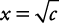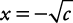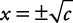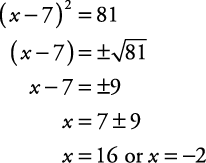## Solving Quadratics by the Square Root Property

The square root property says that if x 2 = c, thenor. This can be written as “if x 2 = c, then.” If c is positive, then x has two real answers. If c is negative, then x has two imaginary answers.

##### Example 1

Solve each of the following equations.

1. x 2 = 48

2. x 2 = –16

3. 5 x 2 – 45 = 0

4. ( x – 7) 2 = 81

5. ( x + 3) 2 = 24

1.2.3.4.5.